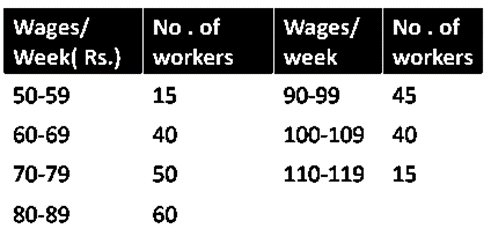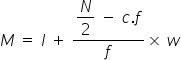Maths-
General
Easy

Question

# The median from the following data is## 83.17    84.08    82.17    85.67Hint:

## The correct answer is: 84.08

### We will use the formula of median to find the answer.From the data, we find the median class. It is range whose cumulative frequency is greater than the total cumulative frequency divided by 2 i.e N/2.N is total cumulative frequency.c.f is the cumulative frequency of a class previous to median class.f is the frequency of the median class.w is the width of the class.l is the lower limit of the median class.Before finding median we will find the cumulative frequency. The frequency of first term is added to the second, then that value is added to next frequency and so on.             Wages No of workers Cummulative Frequency 50 - 59 15  15 60 - 69 40 40 + 15 = 55 70 - 79 50 55 + 50 = 105 80 - 89 60 105 + 60 = 165 90 - 99 45 165 + 45 = 210 100 - 109 40 210 + 40 = 250 110 - 119 15 250 + 15 = 265 So, the value of N here is 265The frequency greater and closest to 132.5 is 165.The median class is 80 - 89l = 80c.f = 105f = 60w = 10Substituting all the values in the formula we get,The value is closest to 84.08So, the right option is 84.08

For such questions, we should know the formula to find a median#### With Turito Foundation.#### Get an Expert Advice From Turito.